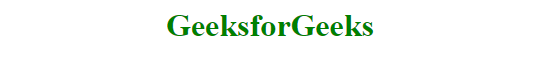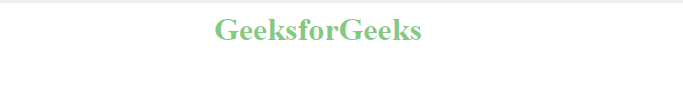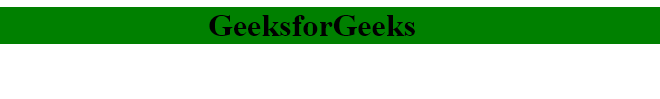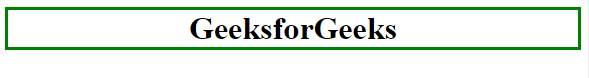Open in App
Not now

# CSS | Colors

• Difficulty Level : Easy
• Last Updated : 03 Dec, 2018

CSS Color property is used to set the color of HTML elements. This property is used to set font color, background color etc.

Color of an element can be defined in the following ways:

• Built-In Color
• RGB Format
• RGBA Format
• HSL
• HSLA

Built-In Color: These are a set of predefined colors which are used by its name. For example: red, blue, green etc.
Syntax:

```h1 {
color: color-name;
}
```

Example:

 `<``html``>``    ``<``head``>``        ``<``title``>CSS color property``        ``<``style``>``            ``h1 {``                ``color:green;``                ``text-align:center;``            ``}``        ````    ````    ``<``body``>``        ``<``h1``>``            ``GeeksforGeeks``        ````    ````                    `

Output:RGB Format: The RGB(Red, Green, Blue) format is used to define the color of an HTML element by specifying the R, G, B values range between 0 to 255. For example: RGB value of Red color is (255, 0, 0), Green color is (0, 255, 0), Blue color is (0, 0, 255) etc.
Syntax:

```h1 {
color: rgb(R, G, B);
}
```

Example:

 `<``html``>``    ``<``head``>``        ``<``title``>CSS color property``        ``<``style``>``            ``h1{``                ``color: rgb(0, 153, 0);``                ``text-align:center;``            ``}``        ````    ````    ``<``body``>``        ``<``h1``>``            ``GeeksforGeeks``        ````    ````                    `

Output:RGBA Format: The RGBA format is similar to the RGB, but the difference is RGBA contains A (Alpha) which specify the transparency of elements. The value of alpha lies between 0.0 to 1.0 where 0.0. represents fully transparent and 1.0 represents not transparent.
Syntax:

```h1 {
color:rgba(R, G, B, A);
}
```

Example:

 `<``html``>``    ``<``head``>``        ``<``title``>CSS RGBA color property``        ``<``style``>``            ``h1 {``                ``color:rgba(0, 153, 0, 0.5);``                ``text-align:center;``            ``}``        ````    ````    ``<``body``>``        ``<``h1``>``            ``GeeksforGeeks``        ````    ````                    `

Output:Hexadecimal Notation: The hexadecimal notation begins with # symbol followed by 6 characters each range from 0 to F. For example: Red #FF0000, Green #00FF00, Blue #0000FF etc.
Syntax:

```h1 {
color:#(0-F)(0-F)(0-F)(0-F)(0-F)(0-F);
}
```

Example:

 `<``html``>``    ``<``head``>``        ``<``title``>CSS hex property``        ``<``style``>``            ``h1{``                ``color:#009900;``                ``text-align:center;``            ``}``        ````    ````    ``<``body``>``        ``<``h1``>``            ``GeeksforGeeks``        ````    ````                    `

Output:HSL: HSL stands for Hue, Saturation, and Lightness respectively. This format uses the cylindrical coordinate system.

• Hue: Hue is the degree of the color wheel. Its value lies between 0 to 360 where 0 represents red, 120 represents green and 240 represents blue color.
• Saturation: It takes percentage value, where 100% represents completely saturated, while 0% represents completely unsaturated (gray).
• Lightness: It takes percentage value, where 100% represents white, while 0% represents black.

Syntax:

```h1 {
color:hsl(H, S, L);
}
```

Example:

 `<``html``>``    ``<``head``>``        ``<``title``>CSS hsl color property``        ``<``style``>``            ``h1{``                ``color:hsl(120, 100%, 30%);``                ``text-align:center;``            ``}``        ````    ````    ``<``body``>``        ``<``h1``>``            ``GeeksforGeeks``        ````    ````                    `

Output:HSLA: The HSLA color property is similar to HSL property, but the difference is HSLA contains A (Alpha) which specify the transparency of elements. The value of alpha lies between 0.0 to 1.0 where 0.0. represents fully transparent and 1.0 represents not transparent.
Syntax:

```h1 {
color:hsla(H, S, L, A);
}
```

Example:

 `<``html``>``    ``<``head``>``        ``<``title``>CSS hsla color property``        ``<``style``>``            ``h1 {``                ``color:hsla(120, 100%, 50%, 0.50);``                ``text-align:center;``            ``}``        ````    ````    ``<``body``>``        ``<``h1``>``            ``GeeksforGeeks``        ````    ````                    `

Output:Text Color: It is used to set the color of the text.
Syntax:

```h1 {
color:color_name;
}
```

Example:

 `<``html``>``    ``<``head``>``        ``<``title``>CSS text color property``        ``<``style``>``            ``h1 {``                ``color:#009900;``                ``text-align:center;``            ``}``        ````    ````    ``<``body``>``        ``<``h1``>``            ``GeeksforGeeks``        ````    ````                    `

Output:Background Color: It is used to set the background color of an HTML element.
Syntax:

```h1 {
background-color:color_name;
}
```

Example:

 `<``html``>``    ``<``head``>``        ``<``title``>CSS background color property``        ``<``style``>``            ``h1 {``                ``background-color:green;``                ``text-align:center;``            ``}``        ````    ````    ``<``body``>``        ``<``h1``>``            ``GeeksforGeeks``        ````    ````                    `

Output:Border Color: It is used to set the border color of an element. Border-style is used to set the border type.
Syntax:

```h1 {
border-style:solid/dashed/dotted
border-color:color_name;
}
```

Example:

 `<``html``>``    ``<``head``>``        ``<``title``>CSS border color``        ``<``style``>``            ``h1 {``                ``border-style:solid;``                ``border-color:green;``                ``text-align:center;``            ``}``        ````    ````    ``<``body``>``        ``<``h1``>``            ``GeeksforGeeks``        ````    ````                    `

Output:List of Colors: Following are the list of few CSS colors.

Color NameHex(RGB)Decimal(RGB)Color
RedFF0000255, 0, 0
PinkFFC0CB255, 192, 203
OrangeFFA500255, 165, 0
YellowFFFF00255, 255, 0
VioletEE82EE238, 130, 238
Green0080000, 128, 0
Aqua00FFFF0, 255, 255
Blue0000FF0, 0, 255
BrownA52A2A165, 42, 42
WhiteFFFFFF255, 255, 255
Gray808080128, 128, 128
Black0000000, 0, 0

My Personal Notes arrow_drop_up